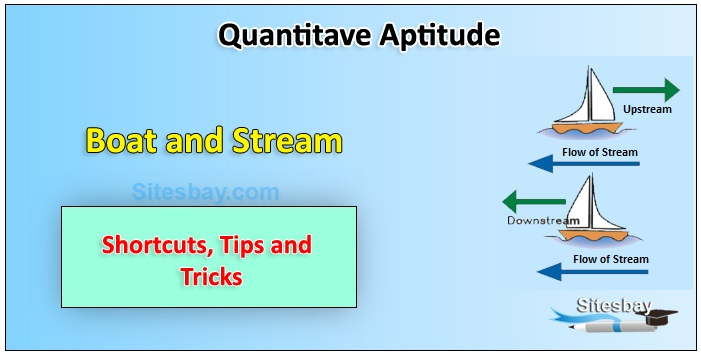# Boat and Stream Problem Concept

## Boat and Stream Problem Tips and Tricks

Still water: The water of a river or any other water body which is not flowing is known as still water.

Stream: It is the flowing water of a river which is moving at a certain speed.

Downstream: In water direction along the stream is called downstream.

Upstream: In water direction against stream is called upstream.Rule: If the speed of a boat in still water is x km/hr and the speed of the stream is y km/hr, then:

## Formula

```
Speed downstream = ( x + y ) km/hr

Speed upstream = ( x - y ) km/hr
```

Rule: If the downstream speed is a km/hr and the speed upstream is b km/hr, then

## Formula

```Speed in Still water = 1/2(a+b)

Rate of Stream = 1/2(a-b)
```

Question 1: A boatman rows his boat 48 km upstream and same distance downstream. The boat takes 12 hrs and 8 hrs to go upstream and downstream respectively. Find the speed of the boat in stagnant water and the speed of the stream respectively.

• (A). 4 km/hr ; 3 km/hr
• (B). 5 km/hr ; 1 km/hr
• (C). 6 km/hr ; 4 km/hr
• (D). 12 km/hr ; 6 km/hr
• (E). Cannot be determined

Answer: (B). 5 km/hr ; 1 km/hr

## Explanation

```Man's/Boat's Speed = X
Stream/Current/River Speed = Y

∴ Downstream speed = X + Y
Upstream speed = X - Y
Downstream Speed = Distance covered/Time taken = 48/8 = 6 km/hr

Upstream Speed = Distance covered/Time taken = 48/12 = 4 km/hr

X+Y = 6 km/hr    and    X-Y = 4 km/hr

X+Y+X-Y = 10 km/hr

∴ X=5 km/hr = Speed of Boat
Y=6-5 = 1 km/hr = Speed of stream
```

Question 2: The time taken by swimmer to swim upstream is 4 hours more than the time he takes to swim downstream. He swims at a speed of 10 km/hr in still water. The stream is flowing gently at 2 km/hr. What is the swimming distance one side?

• (A). 20 km
• (B). 72 km
• (C). 80 km
• (D). 96 km

## Explanation

```Man's/Boat's Speed = X
Stream/Current/River Speed = Y

∴ Downstream speed = X + Y
Upstream speed = X - Y
X+Y = 10+2 = 12 km/hr    and    X-Y = 10-2 = 8 km/hr
Let Time be T hours for downstream
Distance is same
∴ D = D
∴ 12 x T = 8 x (T+4)
∴ T = 8 hours = Time for downstream
Distance = 12km/hr x 8 hours = 96 km
```

Question 3: A motorboat, whose speed in 15 km/hr in still water goes 30 km downstream and comes back in a total of 4 hours 30 minutes. The speed of the stream (in km/hr) is:

• (A). 4
• (B). 5
• (C). 6
• (D). 10

## Explanation

```Let the speed of the stream be x km/hr. Then,

Speed downstream = (15 + x) km/hr,

Speed upstream = (15 - x) km/hr.

30/(15+x) + 30/(15-x) = 9/2
=900/225-x 2 = 9/2
9 x2 = 225
x2 = 25
x = 5 km/hr
```

Question 4: A man can row 15 km /hr in still water. It takes him twice as long to row upstream as to row downstream. Find the rate of stream ?

## Solution

```Let man's rate in upstream be x km/hr, then his rate in downstream is = 2x kmph
rate is still water = 1/2(2x+x) 3x/2 km/hr
3x/2 = 15,
x = 10km/hr

So rate in down stream= 2x= 20 km/hr

Rate of stream = 1/2(20-10) = 5km/hr
```

Question 5: A man can swim 6 km / hr in still water. If river's rate is 2 km / hr, it takes the man 3 hours to row to a place and back. How far is the place ?

## Solution

```Man's rate in upstream=( 6 - 2 ) km/hr = 4 km/hr

Man's stream rate in downstream= ( 6 + 2 ) km/hr = 8 km/hr

Let the distance of the place be x km then,

x/4+x/8 = 3hrs,
x = 8km/hr
```